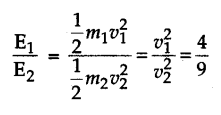# (a) Two bodies have equal masses and are moving with uniform velocities oflv and 3v. What is the ratio of their kinetic energies ? (b) A mass is dropped from height h. At halfway to the ground, what is the total energy?

(a) Given m1 = m,
m2 = m,
v1 = 2v,
v2 = 3v,
EI/E2 = ?
We know that E = 1/2 mv^2, therefore, we have(b) Total energy = KE + PE
Now, velocity after travelling distance h/2 is
v^2 - u^2 = 2g(h/2)
v^2 = gh
Therefore,
KE = 1/2 mv^2 = 1/2 mgh And
PE = 1/2 mgh
Therefore, total energy
= 1/2 mgh + 1/2 mgh = mgh